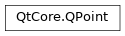# QPoint¶

The `QPoint` class defines a point in the plane using integer precision. More## Detailed Description¶

A point is specified by a x coordinate and an y coordinate which can be accessed using the `x()` and `y()` functions. The `isNull()` function returns `true` if both x and y are set to 0. The coordinates can be set (or altered) using the `setX()` and `setY()` functions, or alternatively the `rx()` and `ry()` functions which return references to the coordinates (allowing direct manipulation).

Given a point p , the following statements are all equivalent:

```p = QPoint()

p.setX(p.x() + 1)
p += QPoint(1, 0)
```

A `QPoint` object can also be used as a vector: Addition and subtraction are defined as for vectors (each component is added separately). A `QPoint` object can also be divided or multiplied by an `int` or a `qreal` .

In addition, the `QPoint` class provides the `manhattanLength()` function which gives an inexpensive approximation of the length of the `QPoint` object interpreted as a vector. Finally, `QPoint` objects can be streamed as well as compared.

`QPointF` `QPolygon`

class PySide2.QtCore.QPoint

PySide2.QtCore.QPoint(QPoint)

PySide2.QtCore.QPoint(xpos, ypos)

param QPoint:

`PySide2.QtCore.QPoint`

param ypos:

int

param xpos:

int

Constructs a null point, i.e. with coordinates (0, 0)

Constructs a point with the given coordinates (`xpos` , `ypos` ).

PySide2.QtCore.QPoint.__reduce__()
Return type:

object

PySide2.QtCore.QPoint.__repr__()
Return type:

object

static PySide2.QtCore.QPoint.dotProduct(p1, p2)
Parameters:
Return type:

int

```QPoint p( 3, 7);
QPoint q(-1, 4);
int lengthSquared = QPoint::dotProduct(p, q);   // lengthSquared becomes 25
```

Returns the dot product of `p1` and `p2` .

PySide2.QtCore.QPoint.isNull()
Return type:

bool

Returns `true` if both the x and y coordinates are set to 0, otherwise returns `false` .

PySide2.QtCore.QPoint.manhattanLength()
Return type:

int

Returns the sum of the absolute values of `x()` and `y()` , traditionally known as the “Manhattan length” of the vector from the origin to the point. For example:

```class MyWidget(QWidget):

self.oldPosition = QPointer()

# event : QMouseEvent
def mouseMoveEvent(QMouseEvent event):
point = event.pos() - self.oldPosition
if (point.manhattanLength() > 3):
# the mouse has moved more than 3 pixels since the oldPosition
pass
```

This is a useful, and quick to calculate, approximation to the true length:

```trueLength = sqrt(pow(x(), 2) + pow(y(), 2))
```

The tradition of “Manhattan length” arises because such distances apply to travelers who can only travel on a rectangular grid, like the streets of Manhattan.

PySide2.QtCore.QPoint.__ne__(p2)
Parameters:
Return type:

bool

PySide2.QtCore.QPoint.__mul__(matrix)
Parameters:
Return type:

`PySide2.QtCore.QPoint`

PySide2.QtCore.QPoint.__mul__(factor)
Parameters:

factor – int

Return type:

`PySide2.QtCore.QPoint`

PySide2.QtCore.QPoint.__mul__(factor)
Parameters:

factor – int

Return type:

`PySide2.QtCore.QPoint`

PySide2.QtCore.QPoint.__mul__(factor)
Parameters:

factor – float

Return type:

`PySide2.QtCore.QPoint`

PySide2.QtCore.QPoint.__mul__(factor)
Parameters:

factor – float

Return type:

`PySide2.QtCore.QPoint`

PySide2.QtCore.QPoint.__mul__(factor)
Parameters:

factor`double`

Return type:

`PySide2.QtCore.QPoint`

PySide2.QtCore.QPoint.__mul__(factor)
Parameters:

factor`double`

Return type:

`PySide2.QtCore.QPoint`

PySide2.QtCore.QPoint.__mul__(m)
Parameters:
Return type:

`PySide2.QtCore.QPoint`

PySide2.QtCore.QPoint.__mul__(matrix)
Parameters:
Return type:

`PySide2.QtCore.QPoint`

PySide2.QtCore.QPoint.__mul__(m)
Parameters:
Return type:

`PySide2.QtCore.QPoint`

PySide2.QtCore.QPoint.__imul__(factor)
Parameters:

factor`double`

Return type:

`PySide2.QtCore.QPoint`

Multiplies this point’s coordinates by the given `factor` , and returns a reference to this point. For example:

```p = QPoint(-1, 4)
p *= 2.5  # p becomes (-3, 10)
```

Note that the result is rounded to the nearest integer as points are held as integers. Use `QPointF` for floating point accuracy.

`operator/=()`

PySide2.QtCore.QPoint.__imul__(factor)
Parameters:

factor – int

Return type:

`PySide2.QtCore.QPoint`

Multiplies this point’s coordinates by the given `factor` , and returns a reference to this point.

`operator/=()`

PySide2.QtCore.QPoint.__imul__(factor)
Parameters:

factor – float

Return type:

`PySide2.QtCore.QPoint`

Multiplies this point’s coordinates by the given `factor` , and returns a reference to this point.

Note that the result is rounded to the nearest integer as points are held as integers. Use `QPointF` for floating point accuracy.

`operator/=()`

Return type:

`PySide2.QtCore.QPoint`

Parameters:
Return type:

`PySide2.QtCore.QPoint`

Parameters:
Return type:

`PySide2.QtCore.QPoint`

Adds the given `point` to this point and returns a reference to this point. For example:

```p = QPoint( 3, 7)
q = QPoint(-1, 4)
p += q    # p becomes (2, 11)
```

`operator-=()`

PySide2.QtCore.QPoint.__sub__()
Return type:

`PySide2.QtCore.QPoint`

PySide2.QtCore.QPoint.__sub__(p2)
Parameters:
Return type:

`PySide2.QtCore.QPoint`

PySide2.QtCore.QPoint.__isub__(p)
Parameters:
Return type:

`PySide2.QtCore.QPoint`

Subtracts the given `point` from this point and returns a reference to this point. For example:

```p = QPoint( 3, 7)
q = QPoint(-1, 4)
p -= q    # p becomes (4, 3)
```

`operator+=()`

PySide2.QtCore.QPoint.__div__(c)
Parameters:

c – float

Return type:

`PySide2.QtCore.QPoint`

PySide2.QtCore.QPoint.__idiv__(divisor)
Parameters:

divisor – float

Return type:

`PySide2.QtCore.QPoint`

Divides both x and y by the given `divisor` , and returns a reference to this point. For example:

```p = QPoint(-3, 10)
p /= 2.5  # p becomes (-1, 4)
```

Note that the result is rounded to the nearest integer as points are held as integers. Use `QPointF` for floating point accuracy.

`operator*=()`

PySide2.QtCore.QPoint.__eq__(p2)
Parameters:
Return type:

bool

PySide2.QtCore.QPoint.setX(x)
Parameters:

x – int

Sets the x coordinate of this point to the given `x` coordinate.

PySide2.QtCore.QPoint.setY(y)
Parameters:

y – int

Sets the y coordinate of this point to the given `y` coordinate.

PySide2.QtCore.QPoint.toTuple()
Return type:

object

PySide2.QtCore.QPoint.transposed()
Return type:

`PySide2.QtCore.QPoint`

Returns a point with x and y coordinates exchanged:

```QPoint{1, 2}.transposed() // {2, 1}
```
PySide2.QtCore.QPoint.x()
Return type:

int

Returns the x coordinate of this point.

`setX()` `rx()`
`setY()` `ry()`